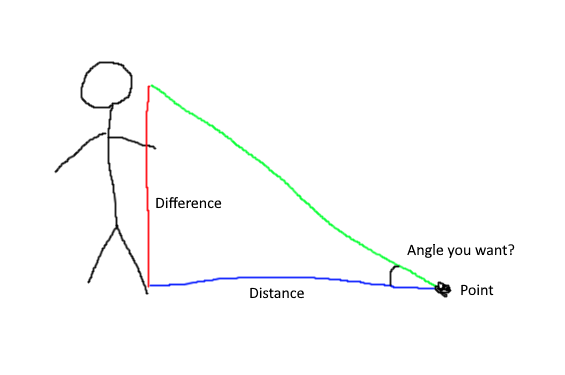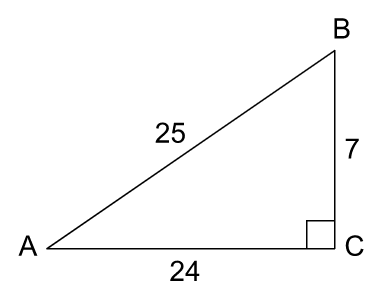Hello,

I was searching for a script that rotate a player’s torso and this one in this topic Need help with torso and head following mouse to where the mouse is positionned but i don’t understand this line here

``````Neck.C0 = Neck.C0:lerp(NeckOriginC0 * CFrame.Angles(-(math.atan(Difference / Distance) * 0.5), (((HeadPosition - Point).Unit):Cross(TorsoLookVector)).Y * 1, 0), 0.5 / 2)
``````

if i understand correctly `Distance` is the Hypotenuse then why he’s dividing Difference/Distance,
in this case he’s caclulating the right angle but when i print the result it shows 45 ,20… degrees

``````local RunService = game:GetService("RunService")

local Player = game.Players.LocalPlayer
local PlayerMouse = Player:GetMouse()

local Camera = workspace.CurrentCamera

local Character = Player.Character or Player.CharacterAdded:Wait()

local Torso = Character:WaitForChild("UpperTorso")
local Waist = Torso:WaitForChild("Waist")

local Humanoid = Character:WaitForChild("Humanoid")
local HumanoidRootPart = Character:WaitForChild("HumanoidRootPart")

local NeckOriginC0 = Neck.C0
local WaistOriginC0 = Waist.C0

Neck.MaxVelocity = 1/3

RunService.RenderStepped:Connect(function()
local CameraCFrame = Camera.CoordinateFrame

local TorsoLookVector = Torso.CFrame.lookVector

if Neck and Waist then
if Camera.CameraSubject:IsDescendantOf(Character) or Camera.CameraSubject:IsDescendantOf(Player) then
local Point = PlayerMouse.Hit.p

local Distance = (Head.CFrame.p - Point).magnitude
local Difference = Head.CFrame.Y - Point.Y

Neck.C0 = Neck.C0:lerp(NeckOriginC0 * CFrame.Angles(-(math.atan(Difference / Distance) * 0.5), (((HeadPosition - Point).Unit):Cross(TorsoLookVector)).Y * 1, 0), 0.5 / 2)
Waist.C0 = Waist.C0:lerp(WaistOriginC0 * CFrame.Angles(-(math.atan(Difference / Distance) * 0.5), (((HeadPosition - Point).Unit):Cross(TorsoLookVector)).Y * 0.5, 0), 0.5 / 2)
end
end
end
end)
````````
1 Like

Rewriting this to not look like a Python programmer touched it:

``````Neck.C0 = Neck.C0:lerp
NeckOriginC0 *
CFrame.Angles
-math.atan(Difference / Distance) * 0.5,
0
0.5 / 2
``````

math.atan finds the angle given the ratio of that angles opposite and adjacent sides (no hypotenuse involved). There is actually a better version, atan2, which also corrects the sign for you:

math.atan2(Distance, Difference)

That said, it looks like you’re trying to do this:3 Likes

This is one script that has been floating around since 2020. It’s not OP’s.

Also how does atan(sin x) give you x …

Infact, I think the original source is from one of my top replies

Code is by CleverSource

1 Like

Distance appears to be the hypotenuse though…

1 Like

It does but if thats the case it shouldn’t be atan.

1 Like

That’s what i am saying but when i test it works perfectly

1 Like

I would definitely agree… but it works though

1 Like

I tried to calculate the right angle of this triangle :using this `math.atan(25/24)` and `math.atan(25/7)` and they both didn’t give me 90 degrees the closest one was 73 degrees it’s kinda weird

1 Like

why would you want to calculate the 90 degrees? you can’t do it using the conventional method of right angled trigonometry. the fact that 25, 24 and 7 are a pythagorian triplet proves it?1 Like

I just found it on a script that i want to use for my game but i didn’t know that calculating the right angle of a right triangle using trigonometry is impossible

1 Like

it’s “impossible” using right angled trigonometry because it would result in a triangle having two 90 degree angles

1 Like

Then how you can do it ? (i will be afk for some time so i might not reply immediatly)

1 Like

there are other methods like the sine rule and the cosine rule etc…

1 Like

Where did you get 50?
123123123123

1 Like

TLDR,math.atan(Adjacent / Hypotenuse) don’t return 90 degrees using trigonometry functions i believe you need to use the law of cosine or the law of sine to achieve this result however i didn’t test that

``````Neck.C0 = Neck.C0:lerp(NeckOriginC0 * CFrame.Angles(-(math.atan(Difference / Distance) * 0.5), (((HeadPosition - Point).Unit):Cross(TorsoLookVector)).Y * 1, 0), 0.5 / 2)
``````

As you may not be familiar with reading one-liners in code, breaking it down step by step can be helpful:

1. `math.atan(Difference / Distance)` — This portion of code calculates an angle based on the vertical difference and distance between the character’s head and the mouse cursor. Specifically, this is the arctangent of the ratio between these values. This angle is used to adjust the character’s head tilt (up or down)
2. `(((HeadPosition - Point).Unit):Cross(TorsoLookVector)).Y` — This part calculates the Y component of the cross product between two vectors: one from the character’s head to the mouse cursor and the other representing the character’s torso look direction. This calculation is used for turning the character’s head left or right.
3. The values `0.5` and `1` — this might be the most confusing part of my code, although it’s quite simple. These constants are multipliers to influence the final rotation. For example, multiplying the arctangent by `0.5` reduces its influence on the overall rotation, which results in a smoother head movement. The `1` multiplier, in this case, was originally to control the sensitivity of the left-right head movement, although I (most) likely realized that it was unnecessary and looked fine without being multiplied. Considering that I wrote this code a few years ago, I’ll assume the latter.
4. `0.5 / 2` — Considering that this is at the end of the `lerp` call, it should be obvious; it controls the speed of interpolation.
``````(((HeadPosition - Point).Unit):Cross(TorsoLookVector)).Y
``````

A deeper dive into this equation might be helpful, although if you don’t have a strong foundation in vector math, you might want to do some research. Here’s the best explanation I can think of step by step:

1. `(HeadPosition - Point).Unit` — This calculates a vector from the character’s head to the point where the mouse intersects the 3D world. The subtraction of these two vectors (`HeadPosition - Point`) results in a vector that points from the head to the cursor’s position. The `.Unit` operation simply normalizes the vector, which means it scales it to have a length ofo 1 while preserving its direction. So this part represents the direction from the head to the cursor.
2. `:Cross(TorsoLookVector)` — This operation crosses the normalized direction vector (which is explained above) with the torso look vector (`TorsoLookVector`). The cross product of these two vectors yields a new vector that is perpendicular to both input vectors. In this case, it’s finding a vector that is perpendicular to both the direction from the head to the cursor and the character’s torso look direction.
3. `.Y` — Finally, we only need the Y component. Why? Because the Y component is the vertical component of the vector, thus, it determines how much the character’s head should be rotated up or down.

I hope this helps and enjoy your day. Thanks!1 Like

This topic was automatically closed 14 days after the last reply. New replies are no longer allowed.